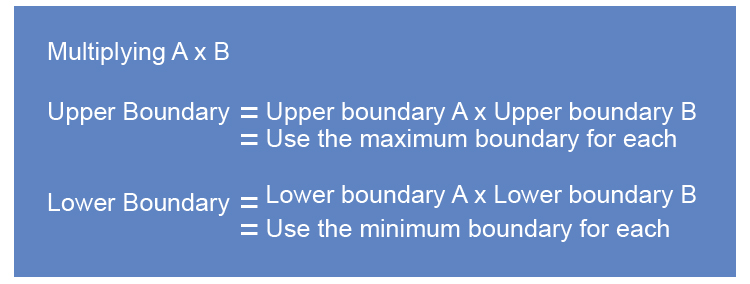# Upper and lower boundary multiplication

If you are looking to multiply two quantities and you need to know the possible limits upper boundary and lower boundary use logic.NOTE:

1. Upper boundary = Upper boundary Atimes Upper boundary B

Multiplying the two largest possible boundaries will provide you with the biggest quantity possible.

Upper boundary        BigtimesBig= Largest amount

2. Lower boundary = Lower boundary Atimes Lower boundary B

Multiplying the two smallest possible lower boundaries will provide you with the smallest quantity possible.

Lower boundary = SmallesttimesSmallest=Smallest amount

Example 1

The area of a floor that needs painting has been measured as 5mtimes4m to the nearest metre. What are the minimum and maximum areas that this could be?

This question is a multiplication question but the first thing you need to do is find the maximum and minimum values of each number.

The Upper boundary and lower boundary for 5m would be:

5m to the nearest 1m

ul5 underline the last significant number.

ul5\.0 look next door

0      5 or more raises the score. So 4.5 would raise to 5.

0       four or less just ignore 5.499 it would ignore and stay at 5.

This is simplified to 5.5

So the upper and lower boundary of 5m is 5.5m and 4.5m.

The upper boundary and lower boundary of 4m would be:

4m to the nearest 1m

ul4 underline the last significant number.

ul4\.0 look next door

0      5 or more raises the score. So 3.5 would raise to 4.

0       four or less just ignore 4.499 it would ignore and stay at 4.

This is simplified to 4.5

So the upper and lower boundary of 4m is 4.5m and 3.5m.

Summary

Upper and lower boundary of 5m is 5.5m and 4.5m

Upper and lower boundary of 4m is 4.5m and 3.5m

So the lowest area dimensions would be the minimum boundary of each which is:

 4.5 xx 3.5 =15.75m^2 Small Small

The highest area dimensions would be the maximum boundaries of each which is:

 5.5 xx 4.5 =24.75m^2 Big Big

Answer: The minimum and maximum this area could be is 15.75m^2 and 24.75m^2.

Example 2

The width of a rectangle was measured as 50cm and was correct to 2 significant figures and the length was measured as 120cm correct to 3 significant figures. What are the lower and upper bound areas that this rectangle could be?

This question is a multiplication question but the first thing you need to do is find the maximum and minimum values of each number.

The Upper boundary and lower boundary for 50cm to 2 significant figures would be:

50cm to 2 significant figures

5ul0 underline the last significant number.

5ul0\.0 look next door

0      5 or more raises the score. So 49.5 would raise to 50.

0       four or less just ignore 50.49dot9 it would ignore and stay at 50.

This is simplified to 50.5

So the upper and lower boundary of 50cm is 50.5cm and 49.5cm.

The upper boundary and lower boundary of 120cm to 3 significant figures would be:

120cm to 3 significant figures.

12ul0 underline the 3^(rd)significant number.

12ul0\.0 look next door

0      5 or more raises the score. So 119.5 would raise to 120.

0       four or less just ignore 120.499 it would ignore and stay at 120.

This is simplified to 120.5

So the upper and lower boundary of 120cm is 120.5cm and 119.5cm.

Summary

Upper and lower boundary of 50cm is 50.5cm and 49.5cm

Upper and lower boundary of 120cm is 120.5cm and 119.5cm

So the lowest area dimensions would be the minimum boundary of each which is:

 49.5 xx 119.5 =5915.25cm^2 to 2 D.P. Small Small

The highest area dimensions would be the maximum boundaries of each which is:

 50.5 xx 120.5 =6085.25cm^2 to 2 D.P. Big Big

Answer: The minimum and maximum this area could be is 5915.25cm^2 and 6085.25cm^2.